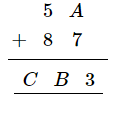# Replace A, B, C by suitable numerals.

Question:

Replace A, B, C by suitable numerals.Solution:

$A=6$

$\therefore \quad A+7=6+7=13$

1 is carried over.

$(1+5+8)=14$

1 is carried over.

$\therefore B=4$

and $C=1$

$\therefore A=6, B=4$ and $C=1$